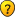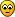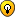## Number of possible 16x16 sudoku grids?

Everything about Sudoku that doesn't fit in one of the other sections

### Number of possible 16x16 sudoku grids?

Has anyone found the exact number of possible 16x16 sudoku grids yet? Not according to wikipedia and OEIS, but that might have changed in the 15 (probably) years since they were last updated. I have also not been able to find anyone doing it online. I know there's a very good estimation out there of around 10^98 though.
yes_maybe

Posts: 3
Joined: 09 January 2020

### Re: Number of possible 16x16 sudoku grids?

The methodology would, I expect, be along similar lines to the original Sudoku 9x9 case. See here for a relatively recent discussion with some useful links.

The Burnside's Lemma approach would seem to be only way forward, since it does not require enumeration of all the grids, but even with this method the numbers are still daunting. This was the method used to count the ED Sudoku 9x9 grids.

To begin with we would need to identify equivalence (conjugacy) classes. The only software for this is a group-theory package like GAP. The number of VPT's (Validity Preserving Transformations) involved, is, I believe, 2 x (12 ^ 10) = 123,834,728,448 for 16x16 Sudoku, compared with just 2 x 6^8 = 3,359,232 for 9x9 Sudoku.

With a group of that size, it's highly unlikely that any desktop PC is going to be able to accomplish even this first step! Some serious architecture would need to be thrown at the problem ...Mathimagics
2017 Supporter

Posts: 1581
Joined: 27 May 2015
Location: Canberra

### Re: Number of possible 16x16 sudoku grids?

Hi, Mathimagics!
I think, yes_maybe says not about essentially different 16 x 16 Sudoku solution grids, but about (simply) different 16 x 16 Sudoku solution grids. It were Bertram Felgenhauer and Frazer Jarvis, who published well-known number of different 9 x 9 Sudokus - 670903752021072936960 (6.671 × 10^21 appox.). Felgenhauer and Frazer Jarvis used as they said "brute force" method to count all 9 x 9 Sudokus, so their method cannot be used for 16 x 16 Sudoku counting. 10^98 is an estimate of different 16 x 16 grids, published at "Mathematics of Sudoku" page of Wikipedia.

As fas as I know, nobody at this forum ever discussed 16 x 16 Sudoku solution grids enumeration.

Serg
Serg
2018 Supporter

Posts: 716
Joined: 01 June 2010
Location: Russia

### Re: Number of possible 16x16 sudoku grids?

Hi Serg,

Serg wrote:As fas as I know, nobody at this forum ever discussed 16 x 16 Sudoku solution grids enumeration.

And we can see why!

For 16x16 Sudoku, assuming that the "different grids" estimate of 5.9584 x 10^98 is a good one, we can divide this by 12! (relabellings) x (2 x 12^10) (VPT's) and get a rough estimate of the number of ED grids, which is 2.3 x 10^74.

The computational challenges to obtaining exact counts (ED or total grids) are plain to see ...Mathimagics
2017 Supporter

Posts: 1581
Joined: 27 May 2015
Location: Canberra

### Re: Number of possible 16x16 sudoku grids?

It’s been done
I’m on holiday in Tenerife can’t find the link !!!!
coloin

Posts: 1915
Joined: 05 May 2005

### Re: Number of possible 16x16 sudoku grids?

Well, thanks for all that. I'm aware of the estimation and the insane computing power required... However, according to Felgenhauer and Jarvis,
Although our original method required a couple of hours of computer time, Guenter Stertenbrink and Kjell Fredrik Pettersen have subsequently developed a method which completes the entire calculation in less than a second!

and that's on a 2006 computer too... Maybe enumeration of 4x4 sudoku grids be doable on a modern computer in a week now?
(I've also found no mention of this method online anywhere except that paper and wikipedia, which did not cite a source)
yes_maybe

Posts: 3
Joined: 09 January 2020

### Re: Number of possible 16x16 sudoku grids?

Okay, I found the fast counting method... http://forum.enjoysudoku.com/fast-3x3-counting-t2995.html

While this does seem quick, I seem to have underestimated the increase in the amount of computation required from 9x9 to 16x16 way, way too much. I blame scientific notation for making giant numbers (almost a googol) seem insignificant...
I guess that's why no one has done this in 15 years.
yes_maybe

Posts: 3
Joined: 09 January 2020

### Re: Number of possible 16x16 sudoku grids?

Hi, coloin!
coloin wrote:It’s been done
I’m on holiday in Tenerife can’t find the link !!!!

Do you mean the link http://forum.enjoysudoku.com/su-doku-s-maths-t44-525.html#p15909?
But PatmaxDaddy posted there an estimate only (formula) for the number of 16 x 16 Sudoku solution grids.

Serg
Serg
2018 Supporter

Posts: 716
Joined: 01 June 2010
Location: Russia

### Re: Number of possible 16x16 sudoku grids?

coloin wrote:Paxmandaddy and kjflp did it

My friend has let sunny (and hopefully, for him, suitably windy) Tenerife go to his headThanks to Serg for correctly identifying the correct name of the contributor (PatmaxDaddy) and the link.

Exact counts require grid enumeration, of some kind, and the numbers are simply too huge for this to be feasible!Mathimagics
2017 Supporter

Posts: 1581
Joined: 27 May 2015
Location: Canberra

### Re: Number of possible 16x16 sudoku grids?

Mathimagics wrote: counts require grid enumeration, of some kind, and the numbers are simply too huge for this to be feasible!

Yes , I apologize for my error, and yes the numbers are probably bigger than too big .

Its not as if we can repeat the 9*9 simulation estimate. [by averaging the no of solutions to a selection of random B1B5B9 and multiplying by 9!^3]

An attempt to do this for the 16x16 with box1,box6,box11,box16 filled in [16!]^4 = 1.9e53 , this would mean that each of these has ~2e45 solutions

Perhaps we could attempt to count the solutions of a completed B1/B6/B11/B16 plus a template of ? clues in the other empty boxes.

At least we could get some additional confirmation of the 5e98 number perhaps
coloin

Posts: 1915
Joined: 05 May 2005

### Re: Number of possible 16x16 sudoku grids?

Revisiting this ....

The estimate is ~ 6e98 and I think this is using a formula ....

and some other numbers
? isnt VPT = 24^4,24^4,24^4,24^4 * 2 = 24^16 *2 = 24233149581890213117952 = 2e22[Correction from below - it is 24^4 * 24^4* 24* 24 *2 = (24^10) *2 = 126806761930752 ~1.2 e14]
16! = 20922789888000 = 2 e13
16!^4 = 1.9 e53

so a typical B1/B6/B12/B16 filling on average might have 2 e 45 solutions ... so that wont be an easy one to compute !!

another way could be count the templates [if that is possible]....[ this was I believe qscgz who turned out aka dukuso estimated the 6e21 number originally]

In the 9*9 sudoku grid, 46656 is the number of ways to have 9 "1"s in a grid
Code: Select all
`941492129            9*9*9*4*4*2*2*1*1 = 46656    [`

17972 is the number of ways to add 2s
it seems to be constant at 17972 although It gets complicated because the the opions for a 2 in Box 2 can either be 4 ways 39 times out of 64 [0.609] or 3 ways 25 times out of 64 [ depending where the 1 is].
Addind a clue to box 6 and box 8 can be either 2 or 1 ways [ never 0] -It doesnt seem possible to make an invalid template with just 2 templates

so the average completion to 17972 could be
.. 8^3, 3.61^2, 1.64^2 = 17972
.. 512 * 13 * 2.7 = 17971.2

anyhow, random simulations generated the ways to fill in successive templates , sometimes it would be zero, but the average PRODUCT did give a good approximation to 6e21.
the invalid templates get made at the 3rd level and shown below with reductions on the 3rd template
Code: Select all
`46656  17972  6121  1848  443  96  24  2  1    PRODUCT   =  1.93617E+2246656  17972  6190  1879  426  96  18  4  1    PRODUCT  =   2.87167E+2246656  17972  6096  1680  392  88  14  2  1    PRODUCT   =  8.29440E+2146656  17972  6021  1784  359  63   4  0  0 `

so
for the 16*16
Code: Select all
`16, 9, 4, 1 9 16, 1, 4 4, 1,16, 9 1  4, 9,16`

we have 16^4 * 9^4 * 4^4 = 110075314176 i think is the first template ?

and i have guess/estimated counts for the next ones
Code: Select all
` 16   16^4*9^4*4^4        = 11007531417615   15^4*8.5^4*3.5^4    ~  39656366213  [ it looks like this one would be constant ?]14   14^4*7.5^4*2.5^4    ~   474807128913   13^4*6.5^4*1.5^4    ~    258102298 `

maybe someone could do a few simulations for these and the remaining templates, is this possible ?
Last edited by coloin on Fri Jul 17, 2020 2:17 pm, edited 7 times in total.
coloin

Posts: 1915
Joined: 05 May 2005

### Re: Number of possible 16x16 sudoku grids?

coloin wrote:? isnt VPT = 24^4,24^4,24^4,24^4 * 2 = 24^16 *2 = 24233149581890213117952 = 2e22I think that the number of VPT's is actually 2 x (24 ^ 10) = 126,806,761,930,752

• band/stack permutations: 24 ^ 2
• rows/cols in band/stack: 24 ^ 8
• transposition: 2

[I see that I gave this count as 2 x 12^10 earlier on in this thread, unaccountably setting 4! = 12 (oops)Nobody picked up on this at the time ....]Mathimagics
2017 Supporter

Posts: 1581
Joined: 27 May 2015
Location: Canberra

### Re: Number of possible 16x16 sudoku grids?

Yes, you are correctExtrapolating , making many assumptions and underestimating it a bit !!!

Code: Select all
`16   16^4*9^4*4^4        = 110075314176   1e1115   15^4*8.5^4*3.5^4    ~  39656366213   3e1014   14^4*7.5^4*2.5^4    ~   4748071289    5e9 13   13^4*6.5^4*1.5^4    ~    258102298    2e812   12^4*5.5^4*1              18974736    2e7     6011   11^4*4.5^4*1               6003725    6e610   10^4*3.5^4*1               1500625    1e6 9    9^4*2.5^4*1                256289    3e5     18 8    8^4*1.5^4*1                 20736    2e4 7    7^4*1*1                      2401    2e3 6    6^4*1*1                      1296    1e3 5    5^4*1*1                       625    6e2     24 4    4^4*1*1                       256    3e2 3    3^4*1*1                        81    8e2     2    2^4*1*1                        16    1e2     24 1    1                               1                                          -----                                           e80  *  6e5   = 6 e 85                                            `

Presumably the overall average count for each template could be obtained, as my approximations have been too harsh ....!!
coloin

Posts: 1915
Joined: 05 May 2005

### Re: Number of possible 16x16 sudoku grids?

Well ... the 16*16 is quite a challange .....and incomprehensible numbers ....

so going back to the 9*9 - some time ago
from apparently fruitless discovery

red ed wrote:...... I worked out a while back that there are

T(0) = 1 way of laying down no digits at all
T(1) = 46656 ways of laying down all the 1s
T(2) = 838501632 ways of laying down all the 1s,2s
T(3) = 5196557037312 ways of laying down all the 1s,2s,3s
T(4) = 9631742544322560 ways of laying down all the 1s,2s,3s,4s

and then I ran out of patience, though it's obvious that the sequence ends

T(8) = 6670903752021072936960 ways of laying down all the 1s-8s
T(9) = 6670903752021072936960 ways of laying down all the 1s-9s

I was hoping at the time to see a nice rate of decay in the ratio of T(k)/T(k-1)
-- because there is a decreasing amount of freedom for the choice of 9-cell template as you move through the digits
-- but it wasn't looking very tidy so I gave up. My numbers could be wrong, though.
[EDIT: in fact, they were (thanks Condor for spotting this). I've corrected T(3) and probably T(4) now.]

If these figures are correct , we can get an exact figure and average for the valid ways to complete the 2nd 3rd and 4th templates
Code: Select all
`   46656                                                               838501632 / 46656                     =  17972                                           5196557037312 / 838501632             =  6197.4                                  9631742544322560 / 5196557037312      =  1853.5                         `

From the original approximation by dukuso
Code: Select all
`46656  17972  6121  1848  443  96  24  2  1    PRODUCT   =  1.93617E+2246656  17972  6190  1879  426  96  18  4  1    PRODUCT   =  2.87167E+2246656  17972  6096  1680  392  88  14  2  1    PRODUCT   =  8.29440E+2146656  17972  6021  1784  359  63   4  0  0 `

from 4000 valid grids
Code: Select all
`I deleted 5 of the templates  and the average grid solution count was      698919.2      [160800  -  2872800]I deleted 4 of the templates  and the average grid solution count was        1790.5      [96 - 10944] I deleted 3 of the templates  and the average grid solution count was          25.46     [6 - 264]I deleted 2 of the templates  and the average grid solution count was           2.29     [2,4 or 8]`

Code: Select all
`T(1) =      46656                                               = 46656                                    T(2) =      838501632 / 46656                                   = 17972                                    T(3) =      5196557037312 / 838501632                           =  6197.4                                   T(4) =      9631742544322560 / 5196557037312                    =  1853.5                                   T(5) =    * 3756379592285798400 / 9631742544322560              = * 390       =      698919.2/1790.5   templates can be added    T(6) =    * 264073485337691627520 / 3756379592285798400         = *  70.3     =        1790.5/25.46    templates can be added   T(7) =    * 2931215687248377065472 / 264073485337691627520      = *  11.1     =          25.46/2.29    templates can be added     T(8) =    * 6712483923798783479930 / 2931215687248377065472     = *   2.29    =           2.29/1       templates can be added   T(9) =    * 6712483923798783479930 / 6712483923798783479930     =     1           * ~ estimated `

Code: Select all
`46656  * 17972  *  6197.4 *  1853.5  *  698919  =  6731825925247620121267     6.7e2146656*17972*6197.4*1853.5*390*70.3*11.1*2.29*1  =  6712501929875011822517     6.7e21`

I am not sure if i have just made the numbers fit here, but certainly the 698919 generated from simulations is of the right order

Now the problem we have now is applying this to the much bigger task !
If we could get an estimate of the average number of possibilities for each box at each template level ... we could estimate the average number of templates which one can add to each T
In the 16*16 case we can fix the 1s in B1B2B6 ... depending where you put the 2s in B1 and B6 there are 8 or 9 possible placements of a 2 in B2 ...
I have a shot at it but am checking it
Code: Select all
`+----+----+--|12..|xxxx||....|1**x||....|***x||....|***x|+----+----+--|....|....||....|.1..||....|...2||....|....|+----+----+--  example of 8 ways to insert a 2 in box 2|    |    |`
coloin

Posts: 1915
Joined: 05 May 2005

### Re: Number of possible 16x16 sudoku grids?

I do have one figure worked out, namely the number of different row 2 completions for any given row 1:

There are 748,521 ways to select the composition of the 4 minirows in row 2, and for each composition there are 24^4 permutations, which gives us

• 748,521 x 24^4 = 248,341,303,296Mathimagics
2017 Supporter

Posts: 1581
Joined: 27 May 2015
Location: Canberra